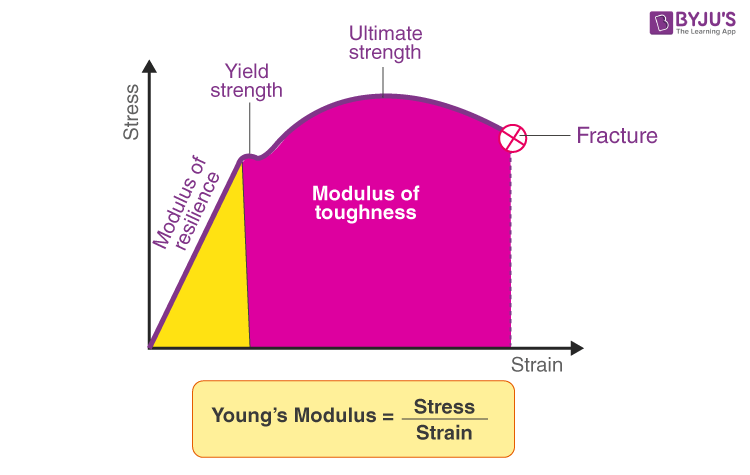# Young's Modulus

In this article, let us learn about modulus of elasticity along with examples. Modulus of elasticity is the measure of the stress–strain relationship on the object. Modulus of elasticity is the prime feature in the calculation of the deformation response of concrete when stress is applied.

Elastic constants are those constants which determine the deformation produced by a given stress system acting on the material. There are many types of elastic constants, like:

• Bulk modulus (K)
• Young’s modulus or modulus of Elasticity (E)
• Poisson’s Ratio (µ)
• Shear modulus or modulus of rigidity (G)

Let us now learn about Young’s modulus, its formula, unit and dimension along with examples. We shall also learn the modulus of elasticity of steel,  glass, wood and plastic.

## What is Young’s Modulus?

Young’s modulus is also known as modulus of elasticity and is defined as:

The mechanical property of a material to withstand the compression or the elongation with respect to its length.

It is denoted as E or Y.

Young’s Modulus (also referred to as the Elastic Modulus or Tensile Modulus), is a measure of mechanical properties of linear elastic solids like rods, wires, and such. There are other numbers that give us a measure of elastic properties of a material, like Bulk modulus and shear modulus, but the value of Young’s Modulus is most commonly used. This is because it gives us information about the tensile elasticity of a material (ability to deform along an axis).

Young’s modulus describes the relationship between stress (force per unit area) and strain (proportional deformation in an object). The Young’s modulus is named after the British scientist Thomas Young. A solid object deforms when a particular load is applied to it. If the object is elastic, the body regains its original shape when the pressure is removed. Many materials are not linear and elastic beyond a small amount of deformation. The constant Young’s modulus applies only to linear elastic substances.

## Young’s Modulus Formula

 $$E=\frac{\sigma }{\epsilon }$$

### Young’s Modulus Formula From Other Quantities

 $$E\equiv \frac{\sigma (\epsilon )}{\epsilon }=\frac{\frac{F}{A}}{\frac{\Delta L}{L_{0}}}=\frac{FL_{0}}{A\Delta L}$$

#### Notations Used In The Young’s Modulus Formula

• E is Young’s modulus in Pa
• 𝞂 is the uniaxial stress in Pa
• ε is the strain or proportional deformation
• F is the force exerted by the object under tension
• A is the actual cross-sectional area
• ΔL is the change in the length
• L0 is the actual length

## Units And Dimension

 SI unit Pa Imperial unit psi Dimension ML-1T-2The Young’s Modulus of such a material is given by the ratio of stress and strain, corresponding to the stress of the material. The relation is given below.

$$E$$ = $$\frac{\sigma}{\epsilon}$$
Where,

• E is the Young’s Modulus of the material given in N/m2
• $$\sigma$$ is the stress applied to the material
• $$\epsilon$$ is the strain corresponding to applied stress in the material

With the value of Young’s modulus for a material, the rigidity of the body can be determined. This is because it tells us about the body’s ability to resist deformation on the application of force.

The Young’s Modulus values $$(x 10^{9} N/m^{2})$$ of different material are given below:

• Steel – 200
• Glass – 65
• Wood – 13
• Plastic (Polystyrene) – 3

## Young’s Modulus Factors

By understanding the modulus of elasticity of steel, we can claim that steel is more rigid in nature than wood or polystyrene, as its tendency to experience deformation under applied load is less. Young’s modulus is also used to determine how much a material will deform under a certain applied load.

Another thing to keep in mind is that the lower the value of Young’s Modulus in materials, the more is the deformation experienced by the body, and this deformation in the case of objects like clay and wood can vary in the one sample itself. One part of the clay sample deforms more than the other whereas a steel bar will experience an equal deformation throughout.

Try calculating the change in length of a steel beam, whose initial length was 200 m, due to applied stress of $$1.5 N/m^{2}$$. Modulus of elasticity of steel can be found in the table above.

## Examples (with solution)

### Example 1

Determine Young’s modulus, when 2 N/m2 stress is applied to produce a strain of 0.5.

Solution:

Given:Stress, σ = 2 N/m2
Strain, ε = 0.5
Young’s modulus formula is given by,
E = σ / ϵ = 2 / 0.5 =4 N/m2

### Example 2

Determine Young’s modulus of a material whose elastic stress and strain are 4 N/m2 and 0.15, respectively.

Solution:

Given: Stress, σ = 4 N/m2
Strain, ε = 0.15
Young’s modulus formula is given by,
E = σ / ϵ
E = 4 / 0.15 =26.66 N/m2

### Give examples of dimensionless quantities.

Following are the examples of dimensionless quantities:

• Poison’s ratio
• Strain

### Give an example of a material with the highest elasticity.

Steel is an example of a material with the highest elasticity.

### What is ductility?

Ductility is defined as the property of a material by which the material is drawn to a smaller section by applying tensile stress.

### What is the dimensional formula of Young’s modulus?

The dimensional formula of Young’s modulus is [ML-1T-2].

### What is the SI unit of Young’s modulus?

Pascal is the SI unit of Young’s modulus.

Hope you have understood modulus of elasticity and Young’s modulus in this article. Stay tuned with BYJU’S for more such interesting articles. Also, register to “BYJU’S – The Learning App” for loads of interactive, engaging Physics-related videos and an unlimited academic assist.

Test Your Knowledge On Youngs Modulus Elastic Modulus!